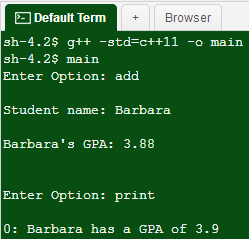# Question & Answer: Background: C++…..

Background: C++

Don't use plagiarized sources. Get Your Custom Essay on
GET AN ESSAY WRITTEN FOR YOU FROM AS LOW AS \$13/PAGE

Programming Language: C++

In this lab you will allocate and free memory in a simple program where you can add or drop students from a class. We supply code for a simple student class with data members name and GPA. In main we declare a vector of pointers to the student class. You will fill in two sections of code.

1) You will define a student member function called ToString which returns a string containing basic student information. For a student named Jane with a GPA of 3.76 the string returned would be:

```Jane has a GPA of 3.8
```

You will create the string using an ostringstream from sstream. Note that you need to setprecision to 1 for that stream.

2) You will write the main function as specified below.

Specification for main – “Add and Drop”

Implement a looping menu which has the options shown in the example below (add, drop, print, and quit). We assume throughout this program that users will always enter reasonable valid inputs, and thus you do not need to test for inappropriate input. Of course, in real code, we would never assume that, but since you have tested inputs many times in other labs, we make this simplifying assumption.

Query a name (assume a one-word name) and GPA and create a new student object (using “new”). Then add the pointer to that object to the students vector.

Print

Print each student using the “ToString” method for the basic student info. For each student also print an index number as shown below.

Drop student

Query an index number and erase the corresponding student pointer from the students vector. Before you erase the pointer you must delete the actual object in memory (using “delete”). Otherwise your program will have a memory leak.

Remember that your vector of pointers resides together on the stack of your main function. However, the objects you create with “new,” and point to from your vector, reside in arbitrary locations on the heap. Thus, it is essential that you free up the object memory in the heap with “delete.”

Execution Example

```Enter Option: add
Student name: Barbara
Barbara's GPA: 3.88

Student name: George
George's GPA: 3.414

Enter Option: print
0: Barbara has a GPA of 3.9
1: George has a GPA of 3.4

Enter Option: drop
Index of student to drop: 1

Enter Option: print
0: Barbara has a GPA of 3.9

Enter Option: quit
```

Given code:

#include <iostream>

#include <string>

#include <vector>

#include <sstream>

#include <iomanip>

using namespace std;

class Student {

public:

Student(string name = “not intialized”, double GPA = 0.0);

void SetName(string studentName);

void SetGPA(double personName);

string ToString() const;

private:

string name;

double GPA;

};

Student::Student(string initialName, double initialGPA) {

name = initialName;

GPA = initialGPA;

}

void Student::SetName(string inputName) {

name = inputName;

}

void Student::SetGPA(double newGPA) {

GPA = newGPA;

}

//Insert the ToString member function here

int main() {

vector<Student*> students;

//Insert your code for main here

return 0;

}

```#include <iostream>
#include <string>
#include <vector>
#include <sstream>
#include <iomanip>

using namespace std;

class Student {
public:
Student(string name = "not intialized", double GPA = 0.0);
void SetName(string studentName);
void SetGPA(double personName);
string ToString() const;
void PrintItem();
private:
string name;
double GPA;
};

Student::Student(string initialName, double initialGPA) {
name = initialName;
GPA = initialGPA;
}

void Student::SetName(string inputName) {
name = inputName;
}

void Student::SetGPA(double newGPA) {
GPA = newGPA;
}

//Insert the ToString member function here FIXME**********************************
string Student::ToString() const {
string AllStudentInfo = "";
ostringstream StudentInfoOSS;

return AllStudentInfo;
}

void Student::PrintItem() {
cout << ": " << this->name << " has a GPA of " << fixed << setprecision(1)
<< this->GPA << endl;
return;
}
//**********************************************************************************
void PrintAllItems(vector<Student*> students) { //FIXME
int unsigned i = 0; // Loop index

// For each item call class member function to print
for (i = 0; i < students.size(); ++i) {
cout << i;
(*students.at(i)).PrintItem();
}
return;
}

int main() {
vector<Student*> students;

Student* newStudent = 0;
Student* tmp = 0;

//Insert your code for main here
int runProgram = 1;
string userOption = "";
string studentName = "";
double studentGPA = 0.0;
int listPosition = 0;

while (runProgram == 1) {

cout << "Enter Option: ";
cin >> userOption;
cout << endl;

if (userOption == "quit") {
runProgram = 0;
}

cout << "Student name: ";
cin >> studentName;
cout << endl;
cout << studentName << "'s GPA: ";
cin >> studentGPA;
cout << endl;
cout << endl;

newStudent = new Student(studentName, studentGPA);
students.push_back(newStudent);
}

if (userOption == "drop") {
cout << "Index of student to drop: ";
cin >> listPosition;
cout << endl;
cout << endl;

tmp = students.at(listPosition);
delete tmp;
students.erase(students.begin() + listPosition);

}

if (userOption == "print") {
PrintAllItems(students);//FIXME
}

}

return 0;
}
```